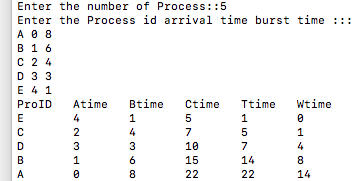# Implementation of Shortest Job First (SJF) Preemptive CPU scheduling algorithm using C++

In this article, we are going to learn about implementation of shortest job first (SJF) preemptive scheduling algorithm using C++ program.
Submitted by Aleesha Ali, on January 29, 2018

Preemptive: If a process of higher priority comes then first CPU will be assign to the Process with higher priority first.

Scheduling criteria tells us that any algorithm is how much efficient, the main criteria of scheduling are given below:

• CPU Utilization
• Throughput
• Arrival time
• Turnaround time
• Waiting time
• Completion time

* Ready Queue is a queue where all the processes wait to get CPU for its execution.

CPU Utilization: The amount of time CPU is busy.

Throughput: The number of process computed per unit time.

Arrival time: The time at which the process enters into ready queue.

Turnaround time: The interval between the times of submission of a process to the time of completion.

Waiting time: The total amount of the time a process spends in ready queue.

Completion time: The time at which process completes its execution.

Burst time: The time needed by CPU to complete its execution.

## SORTEST JOB FIRST (Preemptive)

This algorithm also known as SRTF (Shortest Remaining Time First). As this algorithm is preemptive so the process having minimum arrival time will execute first and after this normal SJF (SHORTEST JOB FIRST) will be follow.

## C++ Program for SJF (Preemptive) scheduling:

```//Implementation fo SHORTEST JOB FIRST(Preemptive) Using C++
#include <iostream>
#include <algorithm>
#include <cstring>
using namespace std;

typedef struct proccess
{
int at,bt,ct,ta,wt,btt;
string pro_id;

/*
artime = Arrival time,
bt = Burst time,
ct = Completion time,
ta = Turn around time,
wt = Waiting time
*/

}Schedule;

bool compare(Schedule a,Schedule b)
{
return a.at<b.at;

/* This Schedule will always return TRUE
if above condition comes*/
}
bool compare2(Schedule a,Schedule b)
{
return a.bt<b.bt;

/* This Schedule will always return TRUE
if above condition comes*/
}

int main()
{
Schedule pro;
//An array of Processes
int n,i,j,pcom;
//n = number of processes, i= iteration variable

cout<<"Enter the number of Process::";
cin>>n;

cout<<"Enter the Process id arrival time burst time :::";

for(i=0;i<n;i++)
{
cin>>pro[i].pro_id;
cin>>pro[i].at;
cin>>pro[i].bt;
pro[i].btt=pro[i].bt;
}

sort(pro,pro+n,compare);

/*sort is a predefined funcion  defined in algorithm.h header file,
it will sort the processes according to their arrival time*/

i=0;
pcom=0;
while(pcom<n)
{
for(j=0;j<n;j++)
{
if(pro[j].at>i)
break;
}

sort(pro,pro+j,compare2);

/*sort is a predefined funcion  defined in algorithm.h header file,
it will sort the processes according to their burst time*/

if(j>0)
{

for(j=0;j<n;j++)
{
if(pro[j].bt!=0)
break;
}
if(pro[j].at>i)

{
i=pro[j].at;

}
pro[j].ct=i+1;
pro[j].bt--;
}
i++;
pcom=0;
for(j=0;j<n;j++)
{
if(pro[j].bt==0)
pcom++;
}
}

cout<<"ProID\tAtime\tBtime\tCtime\tTtime\tWtime\n";

for(i=0;i<n;i++)
{
pro[i].ta=pro[i].ct-pro[i].at;
pro[i].wt=pro[i].ta-pro[i].btt;

/*Printing the Process id, arrival time, burst time,
completion time, turn around time, waiting time*/

cout<<pro[i].pro_id<<"\t"<<pro[i].at<<"\t"<<pro[i].btt<<"\t"<<pro[i].ct<<"\t"<<pro[i].ta<<"\t"<<pro[i].wt;
cout<<endl;
}
return 0;
}
```

Output